Field Theory - Online Tutorial 2

by Kevin Tang , last update: Apr 27, 2014, 2:00PM

[main pages]

ELE401 Final Exam Review Notes (My own note, it have some important formulas that you should know for your final exam) [3.65MB] .. [ Download Here ]

A Letter From Kevin :D

Q1 -

A infinite filaments is placed on x-axis.  (as shown in Figure #1).  It carries current I = 2[A] in negative x-direction.

a) Find the magnetic field intensity vector aΦ at (3, 4, 0).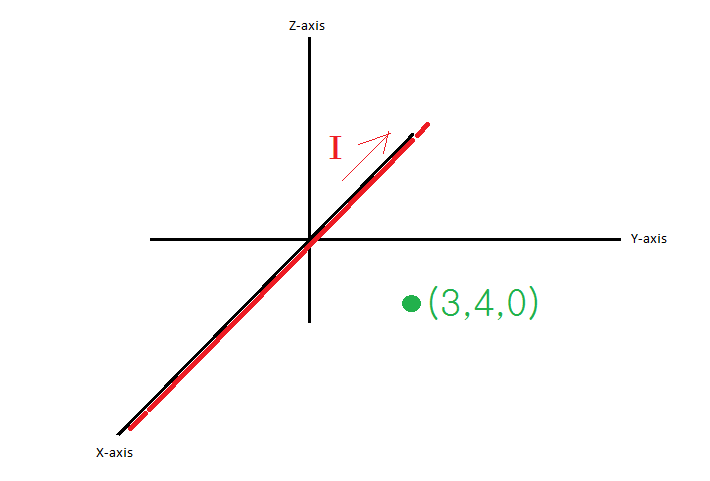Figure #1

Choice one of the following answer.  (Solving Time: 1 min)

SOLUTION 1a)

How to find it?

Simply perform the cross product between the current direction and direct direction.

That is −ax (current direction al) × ay (from the line to the point direction aρ) is equal to −az

aΦ = al × aρ

both al and aρ are in UNIT VECTOR

−az = −ax  × ay

Another way you can find direction as following...

First, put your RIGHT HAND (in case if you don't know which hand is right hand, its the opposite side to your heart *for most people) on

the CURRENT LINE, point your RIGHT HAND THUMB at same direction as the CURRENT GOES

(shown in Figure #2)Figure # 2

as you can see, if you looking from positive X direction toward negative X direction, it is CLOCKWISE

when the FLUX hit the our TARGET POINT (3, 4, 0), its PUSH DOWN toward negative Z (−az)directionFigure # 3

Looking to the Z-Y Plane, you can see it more clear. (shown in Figure #4)Figure # 4

*Important Notice, some case (most case), don't forget the step for unit vector,

our case it cancel out, because √ (32+ 02) = 3 , where 3/3 = 1.

1b)  Now, find H at (3, 4, 0), same graphMaximum Solving Time : 3 min

 A (1/(2π)) (−az) [A/m] Wrong Answer B (1/(4π))  (−az) [A/m] Correct! C (1/π) (−az) [A/m] Wrong Answer D (1/(3π)) (−az) [A/m] Wrong Answer

SOLUTION 1b)

What is the equation for the line current affect on a single point?

The following equation you need to know ... (eq.1)... eq.1

Where, θ1 and θ2 are located as following. (shown in Figure 5)Figure #5

Now, when we have an infinite line, where θ1 will be approach 180o and  θ2 will approach 0o (shown in Figure #6)Figure # 6

Put everything in, you have the equation for Infinite Line (shown in Figure #7)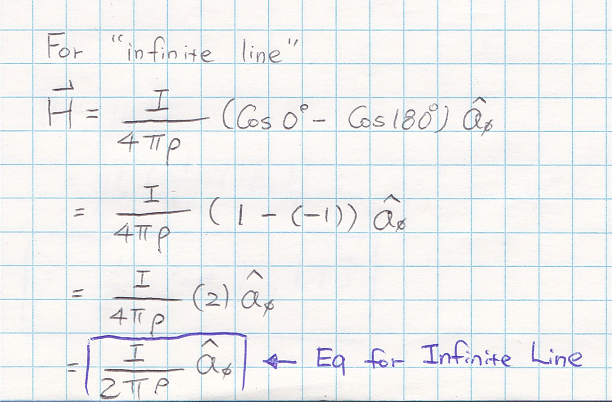Figure #7

With this, your last step is just put everything in,

In our case, its equal to 4 (shown in Figure #8)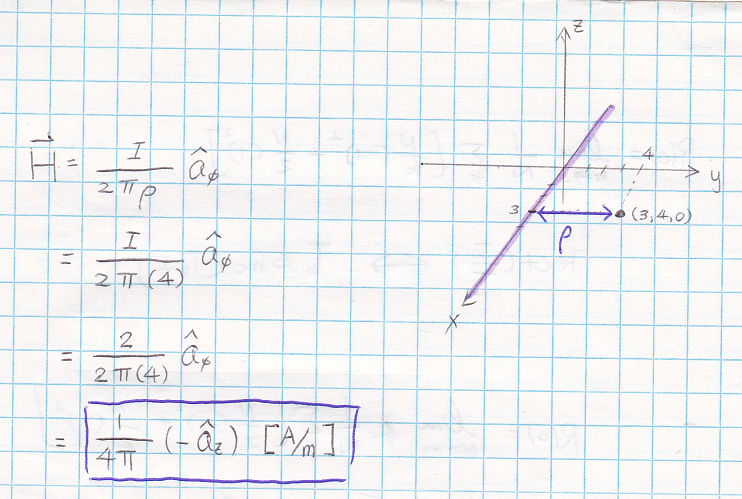Figure #8

Now, you get the final answer -- which is B.

1c) - Given μ = μo, please find B at (3, 4, 0), same graphMaximum solving time: 1 min

 A (μo/(4π)) (−az) [A/m2] Wrong Answer B (μo/(4π)) (−az) [A] Wrong Answer C (2/(μoπ)) (−az) [J/m3] Wrong Answer D (μo/(4π)) (−az) [T] Correct!

This should be very simple, the relation between B and H is as following...

(the way I think, they are brothers or sisters, B is brother for H, as well as D is brother for E)Just multiply it by the constant μo,.. than you get your answer..

The only thing some people may forget is the UNIT for the B, its "Tesla" (T)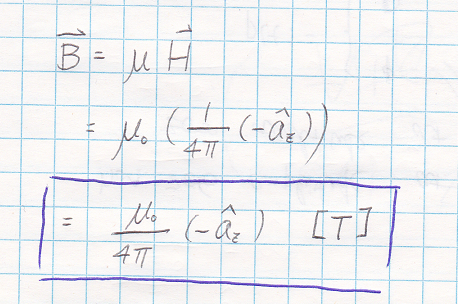So the answer is D, very easy right? lol

* Note: also, T = Wb/m2

Q2 -

Find the work to move the conductor with current I flow in positive az direction,

of the length L1, the conductor is been placed vertically upward at (c, 0, 0),

for one full revolution about the z-axis if the magnetic flux density B = B0ρaρ.

the revolution shape is a circular shape with radius r = c [m].

.

SOLUTION 2)

dF = Idl × B

where dl = dzaz

(notice this dl is the conductor line, with length L1)

Idl × B = I (dzaz × B0ρaρ)

= I B0ρaΦ

notice ... az × aρ = aΦ

dF = I B0ρdz aΦ

than, take integral on both side...

F = ∫0L I B0ρdz aΦ

= L1IB0ρ c aΦ [N]

After you find the force, than

dW = F · dl

where dl = ρdΦaΦ

(This dl is respect to the "one full revolution")

dW = (L1I B0ρ c aΦ) · (ρdΦaΦ)

= L1I B0 c2 dΦ  ... where ρ = c

W = ∫0L1I B0 c2

= 2π L1I B0 c2 [J]

ANSWER: W = 2π L1B0ρ c2 [J]

Q3 - Bio-savart Law question

Base on George's request, this is our question lol

Find H at origin (0, 0, 0), given 2 current line,

Line 1: z = y + 1 (−∞ < y < ∞), current = 2[A] is flowing from negative y direction toward positive y direction.

Line 2 : z = 2y − 4 (0 < y < 2), current = 1[A] is flowing from positive y direction toward negative y direction.

a) Draw the diagram

b) Find H1

c) Find H2

d) Find H

SOLUTION Q3)

To find the H, we can split into H = H1 + H2 , where H1 is the H respect to line 1 and H2 is respect to line 2

First, draw the diagram, you can see the line relationship are all between y and z, so its y-z plan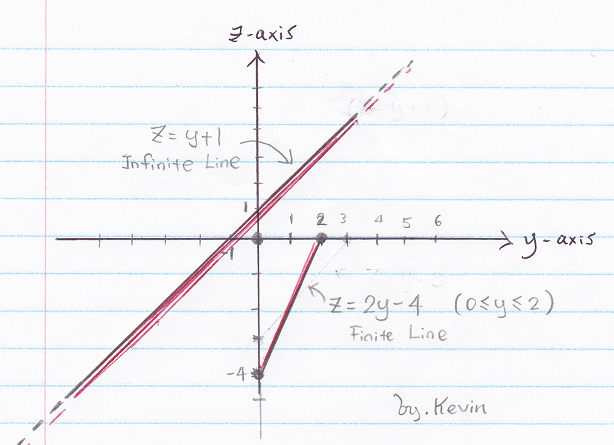looking at line 1 respect to the origin point, use RIGHT HAND RULE, you can see its going toward NEGATIVE X (-ax) DIRECTION,

well, the only issue is you won't able to find the unit vector using right hand rule (but you can use it to check the answer)

the same thing you can applied using equation aΦ = al × aρ

where al is the direction of the current flowing

where aρ is the direction from the LINE to the TARGET POINT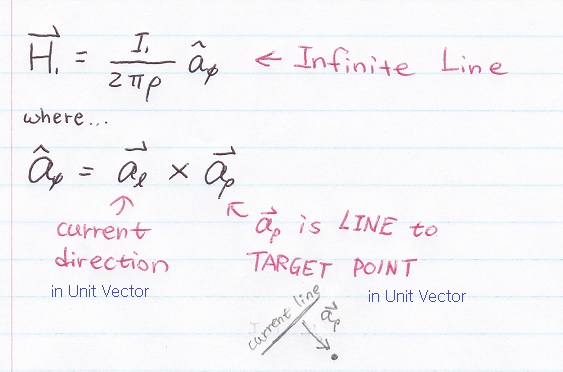ρ is the shortest distance to the extend line (if line is finite, extend the line all the way, ρ will be the shortest distance of this extend line to the point)Next, find H2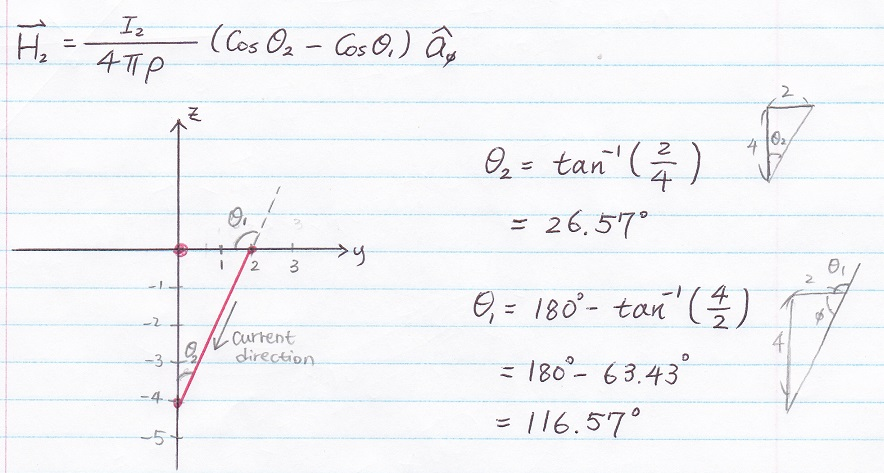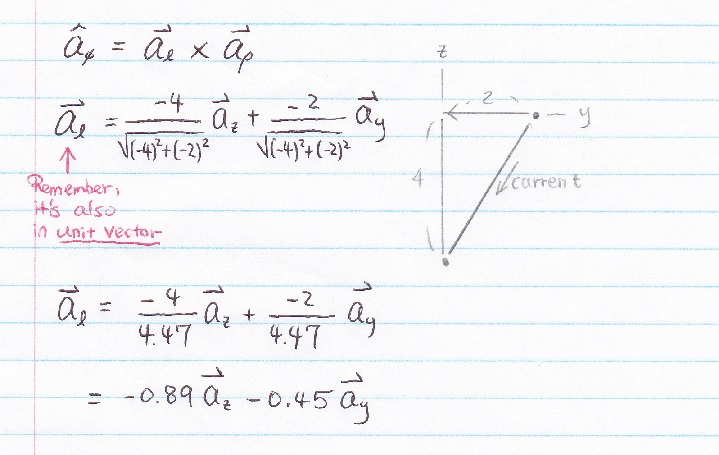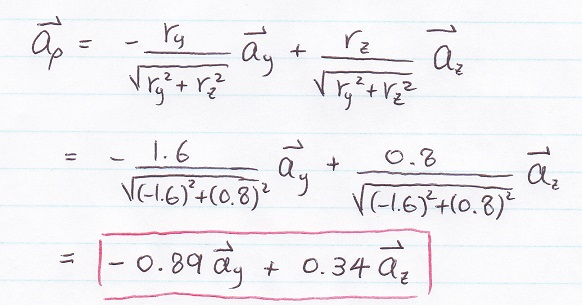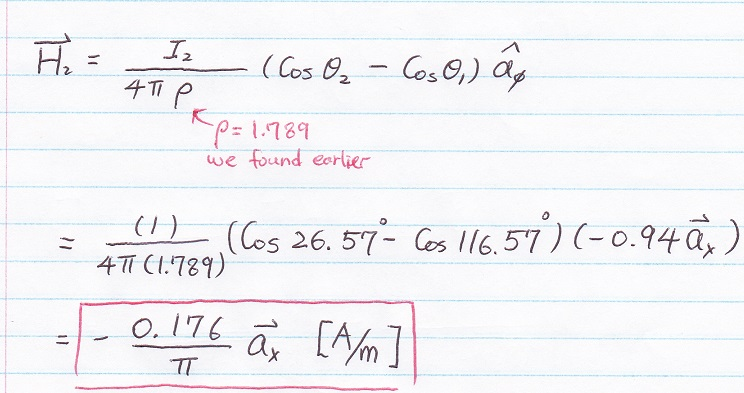Last step... Just add up H1 and H2

H = H1 + H2

*Notice, on the exam, it could be a circular current line instead of straight line...

nothing really change, just use the same concept as you start with

aΦ = al × aρ  the al than will be circular unit vector, the a could be the radius unit vector...

so you cross it, got the result, ... just think more before you start any question

* Question for F (force) and T (torque)

You may leave me any message if you have any questions, I will try to answer ASAP, thanks and good luck :)